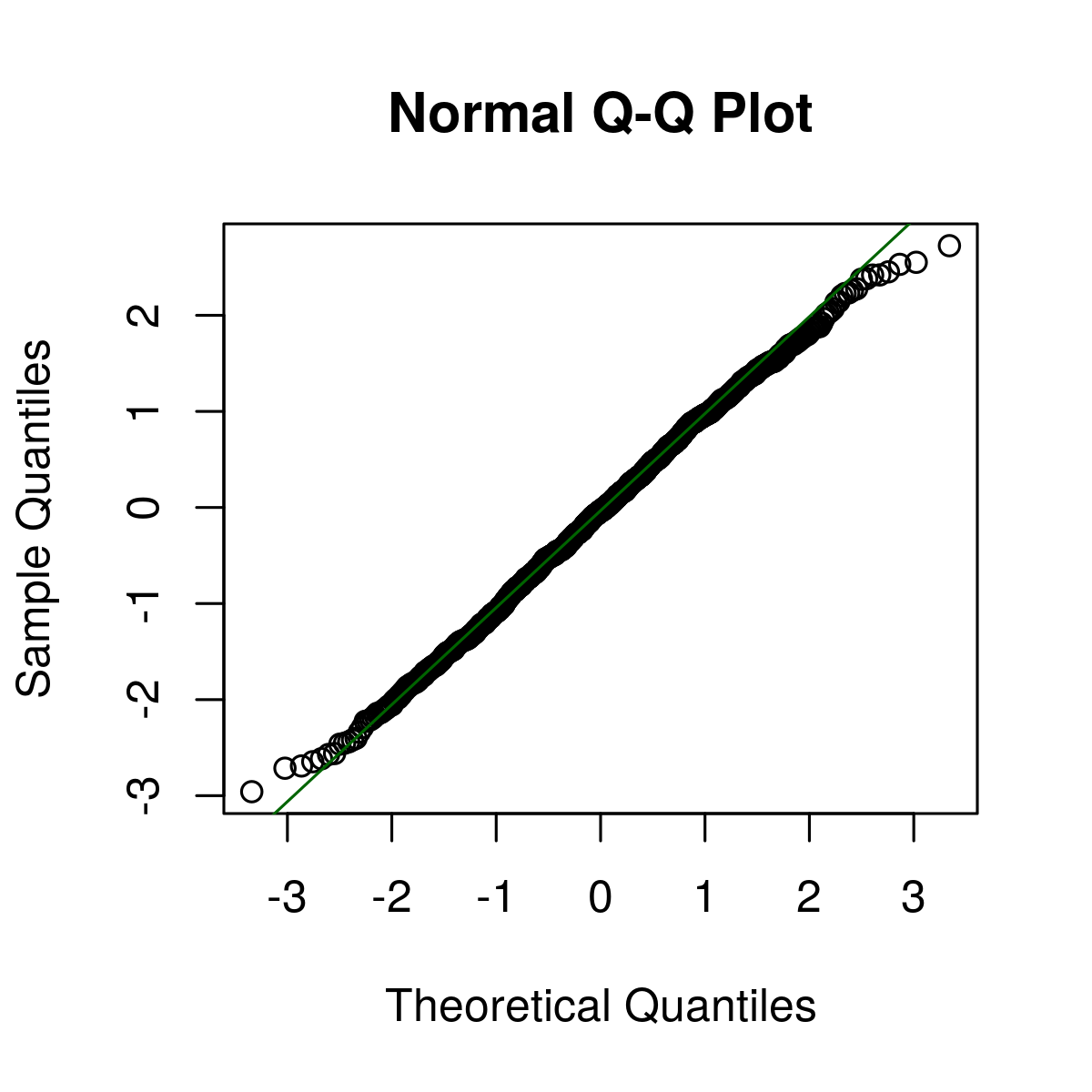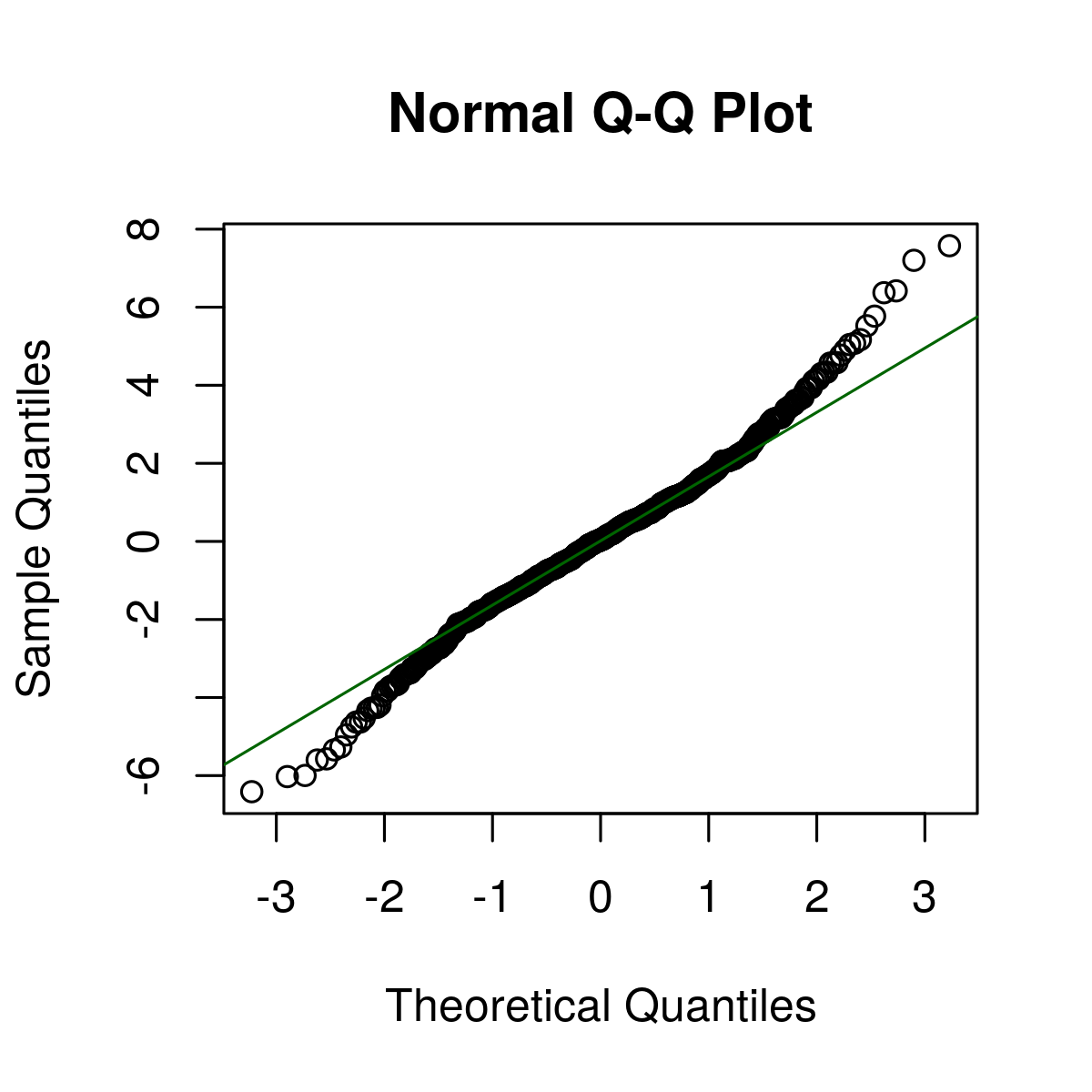# Draw a Quantile-Quantile Plot in R Programming – qqline() Function

The Quantile-Quantile Plot or (Q-Q Plot) is defined as a value of two variables that are plotted corresponding to each other and check whether the distributions of two variables are similar or not with respect to the locations. `qqline()` function in R Language is used to draw a Q-Q Line Plot.

Syntax: qqline(x, y, col)

Parameters:
x, y: X and Y coordinates of plot
col: It defines color

Returns: A QQ Line plot of the coordinates provided

Example 1: Implementation of Basic QQplot Interpretation

 `# Set seed for reproducibility ` `set.seed``(500) ` ` `  `# Create random normally distributed values ` `x <- ``rnorm``(1200) ` ` `  `# QQplot of normally distributed values ` `qqnorm``(x)  ` ` `  `# Add qqline to plot ` `qqline``(x, col = ``"darkgreen"``) `

Output:Above is a representation of QQplot of Normally Distributed Random Numbers.

Example 2: Implementation of QQplot of Logistically Distributed Values

 `# Set seed for reproducibility ` ` `  `# Random values according to logistic distribution ` `# QQplot of logistic distribution ` `y <- ``rlogis``(800)   ` ` `  `# QQplot of normally distributed values ` `qqnorm``(y)  ` ` `  `# Add qqline to plot ` `qqline``(y, col = ``"darkgreen"``) `

Output:Above is the Q-Q Plot of theoretical quantiles.

My Personal Notes arrow_drop_upCheck out this Author's contributed articles.

If you like GeeksforGeeks and would like to contribute, you can also write an article using contribute.geeksforgeeks.org or mail your article to contribute@geeksforgeeks.org. See your article appearing on the GeeksforGeeks main page and help other Geeks.

Please Improve this article if you find anything incorrect by clicking on the "Improve Article" button below.

Article Tags :

Be the First to upvote.

Please write to us at contribute@geeksforgeeks.org to report any issue with the above content.